# Vinegar

We must dilute 16 liters of 8.8% aqueous vinegar to 4.1% one. How much water is necessary to add?

V =  18.3 l

### Step-by-step explanation:

8.8/100 • 16 = (V + 16) • 4.1/100

4.1V = 75.2

V = 752/41 ≈ 18.341463

Our simple equation calculator calculates it.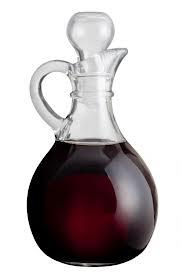Did you find an error or inaccuracy? Feel free to write us. Thank you!Tips to related online calculators
Need help to calculate sum, simplify or multiply fractions? Try our fraction calculator.
Do you have a linear equation or system of equations and looking for its solution? Or do you have a quadratic equation?
Tip: Our volume units converter will help you with the conversion of volume units.

## Related math problems and questions:

• Vinegar 2How many percentages of vinegar solution will we get if we mix 3.5 liters of 5.8% and 5 liters of 7.6% vinegar?
• Concentration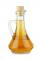How 0.5 liters of 8% vinegar diluted to a concentration of 20 hundredths % of vinegar? How many liters of water must be poured?
• Solutions, mixturesHow many liters of 70% solution we must add to 5 liters of 30% solution to give us a 60% solution?
• Peroxide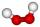How much distilled water (in liters) must pharmacists pour into 300 ml of 23.6% solution of hydrogen peroxide to get 2.7% solution to gargle?
• Sea waterSeawater contains about 4.3% salt. How many dm3 of distilled water we must pour into 5 dm3 of sea water to get water with 1.8% salt?
• AlcoholHow many 55% alcohol we need to pour into 14 liters 75% alcohol to get p3% alcohol? How many 65% alcohol we get?
• Vinegar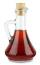What percentage vinegar we get if we mix 1 if dm³ eight percent vinegar with 1.5 dm³ six percent vinegar?
• FertilizerI have 4 liters of 10% fertilizer. How much water do I need to add to have a 2% solution?
• Solutions, mixturesWe have 2 liters of 20% solution available. How much 70% solution do we need to add to it to get a 30% solution?
• Mixing paint with waterMr. Adamek will paint. The purchased paint is diluted with water in a ratio of 1: 1.5. a) how many parts of water will add to 1 part of the paint b) how many liters of water the mission adds to 2 liters of paint
• Vinegar mixing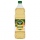We mix 70g water and 50g 8% vinegar. How many percents of the solution of vinegar we produce?
• Pouring alcohol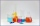100 liters of alcohol has 70% How many liters of water need to be added to have 60% alcohol?
• Mixture 2How many liters of water must be added to 7 liters of a 20% solution to obtain a 10% solution?
• Acid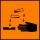The barrel is 199 liters of 38% acid. How many percent acids occur when 61 liters revoke and replace with the same amount of water?
• Disinfecting solutionHow much distilled water is necessary to pour into 500 ml of 33% hydrogen peroxide solution to obtain 3% disinfecting solution?
• Fertilizer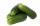Mr. Gherkin use for fertilization 7% solution of fertilizer. 17 liters of it still left. How much water must be added to the solution to make only 3% solution?
• Alcohol mixtureThree liters of 96 percent alcohol set up a certain amount of distilled water to form 54-percent alcohol. How many liters of distilled water was used?